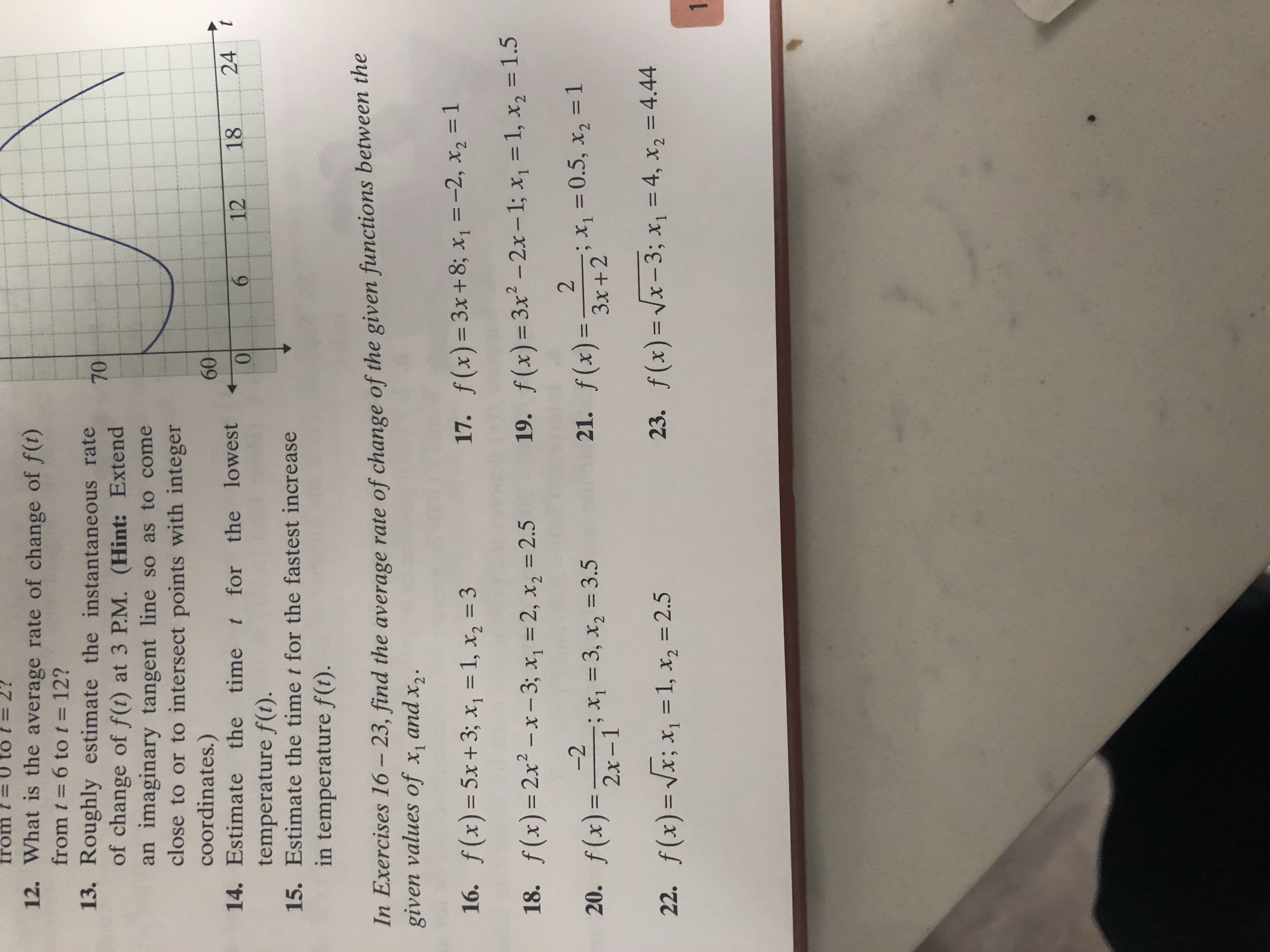# from t = 0 to t = 2? 12. What is the average rate of change of f) from t 6 to t 12? 13. Roughly estimate the instantaneous rate 7 of change of f(t) at 3 P.M. (Hint: Extend an imaginary tangent line so as to come close to or to intersect points with integer coordinates.) temperature f(t). in temperature f(t). 60 14. Estimate the time t for the lowest 6 12 18 24 15. Estimate the time t for the fastest increase In Exercises 16-23, find the average rate of change of the given functions between the 16. f (x)-5x +3, x 1, x2 3 18. f(x) = 2x2-x-3; x1=2, x,-2.5 19. f(x)-3x2-2x-1; x1=1, x2=1.5 20. f(x)--·x,-3, x2-3.5 22, f(x)-./x;, 1, x,-2.5 given values of x, and x 17. f(x) = 3x +8; x1=-2, x,-1 2 2x -1 f(x) = 3x2+2, x,-( .5, x2=1 21. 23. f(x)--/x-3; x1 = 4, x2=4.44

Question

#16 or #20 or both. Thanks confused on how to do them.help_outlineImage Transcriptionclosefrom t = 0 to t = 2? 12. What is the average rate of change of f) from t 6 to t 12? 13. Roughly estimate the instantaneous rate 7 of change of f(t) at 3 P.M. (Hint: Extend an imaginary tangent line so as to come close to or to intersect points with integer coordinates.) temperature f(t). in temperature f(t). 60 14. Estimate the time t for the lowest 6 12 18 24 15. Estimate the time t for the fastest increase In Exercises 16-23, find the average rate of change of the given functions between the 16. f (x)-5x +3, x 1, x2 3 18. f(x) = 2x2-x-3; x1=2, x,-2.5 19. f(x)-3x2-2x-1; x1=1, x2=1.5 20. f(x)--·x,-3, x2-3.5 22, f(x)-./x;, 1, x,-2.5 given values of x, and x 17. f(x) = 3x +8; x1=-2, x,-1 2 2x -1 f(x) = 3x2+2, x,-( .5, x2=1 21. 23. f(x)--/x-3; x1 = 4, x2=4.44 fullscreen

### Want to see the step-by-step answer?